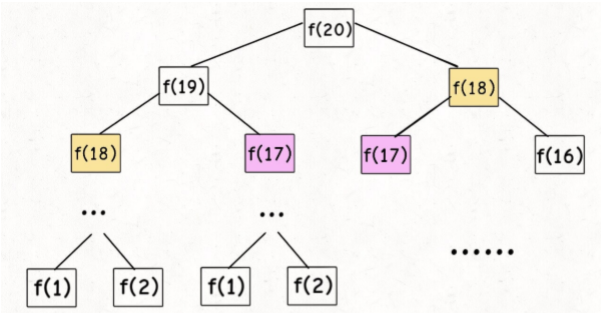## 算法(01)--动态规划详解

### 动态规划详解

⾸先，动态规划的穷举有点特别，因为这类问题存在「重叠⼦问题」，如果 暴⼒穷举的话效率会极其低下，所以需要「备忘录」或者「DP table」来优 化穷举过程，避免不必要的计算。 ⽽且，动态规划问题⼀定会具备「最优⼦结构」，才能通过⼦问题的最值得到原问题的最值。

## 《斐波那契数列》— 主要是让你明⽩什么是重叠⼦问题

### 1.1) 暴⼒递归

``````    int	fib(int	N){

if(N ==	1 || N	==	2) return 1;
return	fib(N-1) + fib(N-2);
}
``````

PS：但凡遇到需要递归的问题，最好都画出递归树，这对你分析算法的复杂度，寻找算法低效的原因都有巨⼤帮助。⼦问题个数，即递归树中节点的总数,显然⼆叉树节点总数为指数级别，所以⼦问题个数为O(2^n)。

### 1.2) 带备忘录的递归解法

⼀般使⽤⼀个数组充当这个「备忘录」，当然你也可以使⽤哈希表（字 典），思想都是⼀样的。

``````    int fib(int N) {

if (N < 1) return 0;
//	备忘录全初始化为0
vector<int> memo (N + 1, 0);
//	初始化最简情况
return helper(memo, N);
}

int helper(vector<int>& memo, int n) {

//	base	case
if (n == 1 || n == 2) return 1;
//	已经计算过
if (memo[n] != 0) return memo[n];
memo[n] = helper(memo, n - 1) + helper(memo, n - 2);
return memo[n];
}
``````⼦问题个数，即图中节点的总数，由于本算法不存在冗余计算，⼦问题就是 f(1) , f(2) , f(3) … f(20) ，数量和输⼊规模 n = 20 成正⽐，所以⼦问题个数为 O(n)。 解决⼀个⼦问题的时间，同上，没有什么循环，时间为 O(1)。 所以，本算法的时间复杂度是 O(n)。⽐起暴⼒算法，是降维打击。
⾄此，带备忘录的递归解法的效率已经和迭代的动态规划解法⼀样了。实际 上，这种解法和迭代的动态规划已经差不多了，只不过这种⽅法叫做「⾃顶向下」，动态规划叫做「⾃底向上」。

### 1.3) dp 数组的迭代解法

``````    int fib(int N) {

vector<int> dp (N + 1, 0);
//	base	case
dp = dp = 1;
for (int i = 3; i <= N; i++)
dp[i] = dp[i - 1] + dp[i - 2];
return dp[N];
}
````````````    int fib(int n) {

if (n == 2 || n == 1) return 1;
int prev = 1, curr = 1;
for (int i = 3; i <= n; i++) {

int sum = prev + curr;
prev = curr;
curr = sum;
}
return curr;
}
``````

## 《凑零钱问题 》— 主要是让你明⽩什么是最优⼦结构

``````//	coins中是可选硬币⾯值，amount是⽬标⾦额
int	coinChange(int[] coins,	int	amount);
``````

⽐如说 k = 3 ，⾯值分别为 1，2，5，总⾦额 amount = 11。那么最少需 要 3 枚硬币凑出，即 11 = 5 + 5 + 1。 你认为计算机应该如何解决这个问题？显然，就是把所有肯能的凑硬币⽅法 都穷举出来，然后找找看最少需要多少枚硬币。

### 2.1) 暴⼒递归

⾸先，这个问题是动态规划问题，因为它具有「最优⼦结构」的。要符合 「最优⼦结构」，⼦问题间必须互相独⽴。啥叫相互独⽴？你肯定不想看数学证明，我⽤⼀个直观的例⼦来讲解。
⽐如说，你的原问题是考出最⾼的总成绩，那么你的⼦问题就是要把语⽂考 到最⾼，数学考到最⾼…… 为了每门课考到最⾼，你要把每门课相应的选择题分数拿到最⾼，填空题分数拿到最⾼…… 当然，最终就是你每门课都是满分，这就是最⾼的总成绩。 得到了正确的结果：最⾼的总成绩就是总分。因为这个过程符合最优⼦结 构，“每门科⽬考到最⾼”这些⼦问题是互相独⽴，互不⼲扰的。

• 先确定「状态」，也就是原问题和⼦问题中变化的变量。由于硬币数量⽆ 限，所以唯⼀的状态就是⽬标⾦额 amount；
• 然后确定 dp 函数的定义：当前的⽬标⾦额是 n，⾄少需要dp(n)个硬 币凑出该⾦额；
• 然后确定「选择」并择优，也就是对于每个状态，可以做出什么选择改变当前状态。具体到这个问题，⽆论当的⽬标⾦额是多少，选择就是从⾯额列表coins中选择⼀个硬币，然后⽬标⾦额就会减少：
``````    //	伪码框架
def coinChange(coins:List[int], amount:int):
//	定义：要凑出⾦额 n，⾄少要dp(n)个硬币
def dp(n):
//	做选择，选择需要硬币最少的那个结果
for coin in coins:
res=min(res,1+dp(n-coin))
return res
//	我们要求的问题是 dp(amount)
return dp(amount)
``````
• 最后明确 basecase，显然⽬标⾦额为 0 时，所需硬币数量为0；当⽬标⾦额⼩于 0 时，⽆解，返回-1：
``````    def coinChange(coins:List[int], amount:int):
def dp(n):
// base case
if n==0:return 0
if n< 0:return-1
// 求最⼩值，所以初始化为正⽆穷
res=float('INF')
for coin in coins:
subproblem=dp(n-coin)
// ⼦问题⽆解，跳过
if subproblem==-1:continue
res=min(res,1+subproblem)
return res if res!=float('INF')else-1
return dp(amount)
``````

⾄此，状态转移⽅程其实已经完成了，以上算法已经是暴⼒解法了，以上代码的数学形式就是状态转移⽅程：⾄此，这个问题其实就解决了，只不过需要消除⼀下重叠⼦问题，⽐如amount = 11, coins = {1,2,5} 时画出递归树看看：⼦问题总数为递归树节点个数，这个⽐较难看出来，是 O(n^k)，总之是指数级别的。每个⼦问题中含有⼀个 for 循环，复杂度为 O(k)。所以总时间复 杂度为O(k * n^k)，指数级别。

### 2.2) 带备忘录的递归

``````        def coinChange(coins:List[int],amount:int):
//	备忘录
memo=dict()
def dp(n):
//	查备忘录，避免重复计算
if n in memo:return memo[n]
if n==0:return 0
if n<	0:return-1
res=float('INF')
for coin in coins:
subproblem=dp(n-coin)
if subproblem==-1:continue
res=min(res,1+subproblem)

//	记⼊备忘录
memo[n]=res if res!=float('INF') else-1
return memo[n]
return dp(amount)
``````

### 2.3) dp 数组的迭代解法

``````        int	coinChange(vector<int>&	coins,	int	amount)	{

//	数组⼤⼩为	amount	+	1，初始值也为	amount	+	1
vector<int>	dp(amount	+	1,	amount	+	1);
//	base	case
dp=0;
for	(int i = 0;	i < dp.size();	i++){

//	内层	for	在求所有⼦问题	+	1	的最⼩值
for	(int coin :	coins)	{

//	⼦问题⽆解，跳过
if	(i - coin <	0)	continue;
dp[i] =	min(dp[i],	1 +	dp[i - coin]);
}
}
return	(dp[amount]	==	amount	+	1)	?	-1	:	dp[amount];
}
``````PS：为啥 dp数组初始化为 amount+ 1 呢？